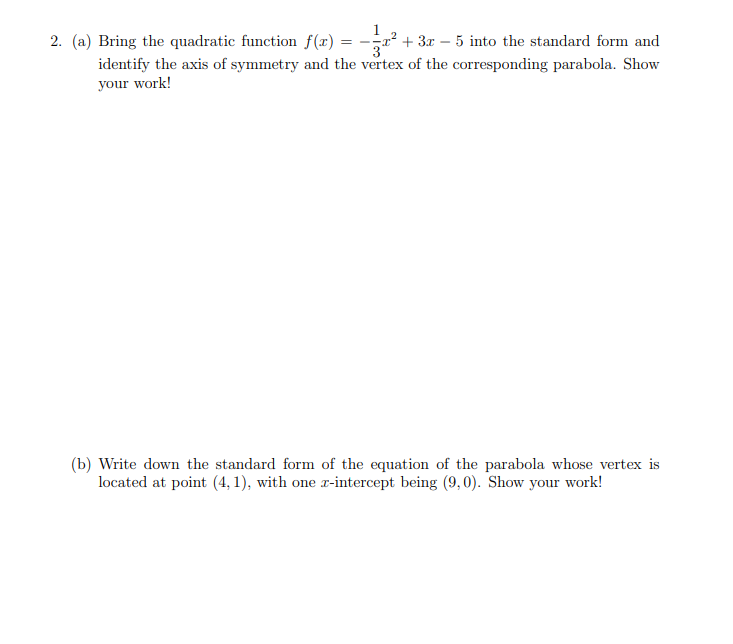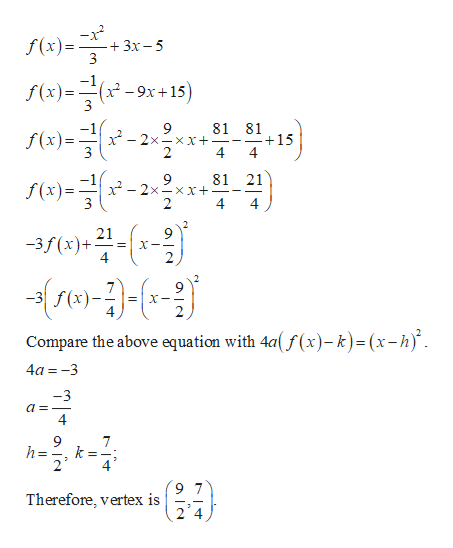# 3 - 5 into the standard form and2. (a) Bring the quadratic function f(x)identify the axis of symmetry and the vertex of the corresponding parabola. Showyour work!(b) Write down the standard form of the equation of the parabola whose vertex islocated at point (4,1), with one x-intercept being (9,0). Show your work!

Question
4 views

question 2help_outlineImage Transcriptionclose3 - 5 into the standard form and 2. (a) Bring the quadratic function f(x) identify the axis of symmetry and the vertex of the corresponding parabola. Show your work! (b) Write down the standard form of the equation of the parabola whose vertex is located at point (4,1), with one x-intercept being (9,0). Show your work! fullscreen
check_circle

Step 1

Consider the given function:

Step 2

Convert the given function in the standard form:help_outlineImage Transcriptionclosef(x)= 3x-5 3 f(x)(29x+ 15) 81 81 +15 4 -2x xx+ 3 4 9 x-2xxx+ 2 81 21 S(x)= 3 4 21 -3f(x)+ X-- 2 re-3- Compare the above equation with 4a( f (x)-k) = (x-h) 4a-3 -3 4 7 k h= Therefore, vertex is fullscreen
Step 3

Consider the information given in th...

### Want to see the full answer?

See Solution

#### Want to see this answer and more?

Solutions are written by subject experts who are available 24/7. Questions are typically answered within 1 hour.*

See Solution
*Response times may vary by subject and question.
Tagged in

### Other